SNOSBJ3E November   1999  – December 2014

PRODUCTION DATA.

1. Features
2. Applications
3. Description
4. Revision History
5. Pin Configuration and Functions
6. Specifications
7. Detailed Description
8. Application and Implementation
1. 8.1 Application Information
2. 8.2 Typical Applications
1. 8.2.1 Basic Comparator
2. 8.2.2 System Examples
9. Power Supply Recommendations
10. 10Layout
11. 11Device and Documentation Support
12. 12Mechanical, Packaging, and Orderable Information

• J|14

## 8 Application and Implementation

NOTE

Information in the following applications sections is not part of the TI component specification, and TI does not warrant its accuracy or completeness. TI’s customers are responsible for determining suitability of components for their purposes. Customers should validate and test their design implementation to confirm system functionality.

### 8.1 Application Information

The LM139-N is specified for operation from 2.0 V to 36 V (±1V to ±18V) over the temperature range of –55°C to 125°C. While it may seem like a comparator has a well-defined and somewhat limited functionality as a '1-bit ADC', a comparator is a versatile component which can be used for many functions.

Refer to AN-74 LM139/LM239/LM339 A Quad of Independently Functioning Comparators (SNOA654) for additional application information on use of the LM139-N.

### 8.2 Typical Applications

#### 8.2.1 Basic Comparator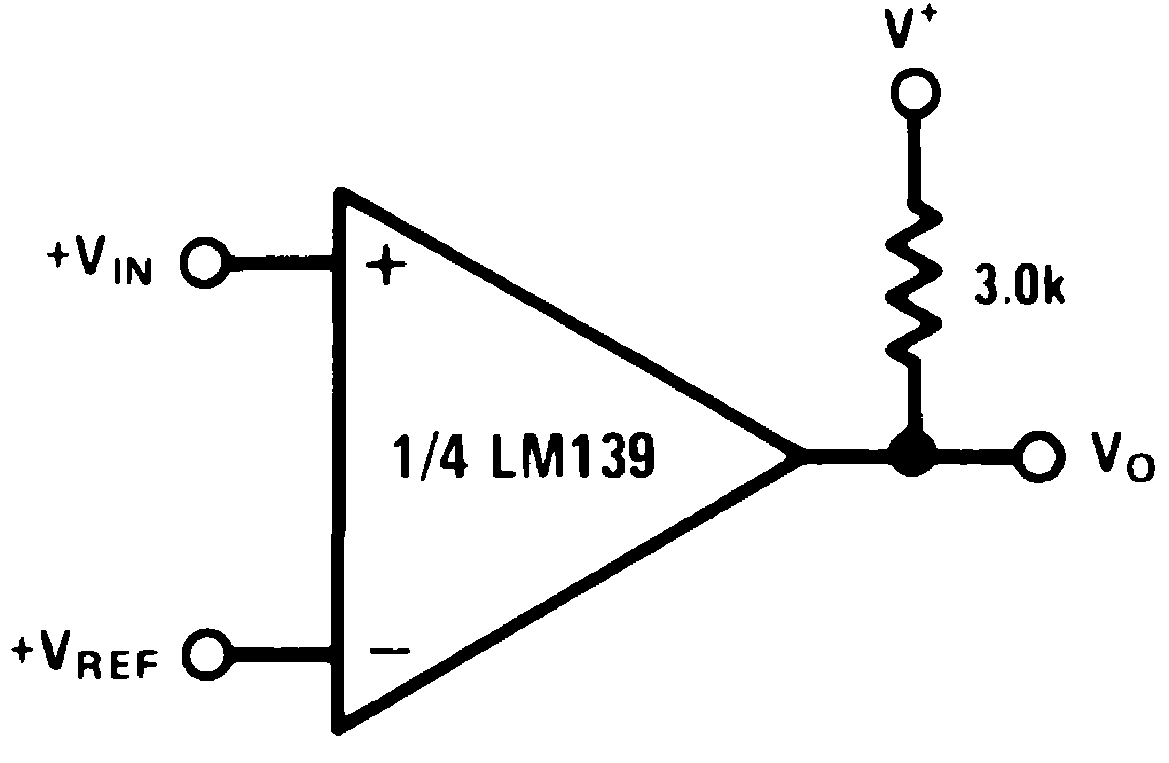Figure 11. Basic Comparator Schematic

#### 8.2.1.1 Design Requirements

The basic usage of a comparator is to indicate when a specific analog signal has exceeded some predefined threshold. In this application, the negative input is tied to a reference voltage, and the positive input is connected to the input signal. The output is pulled up with a resistor to the logic supply voltage, V+.

For an example application, the supply voltage is 5 V. The input signal varies between 1 V and 3 V, and we want to know when the input exceeds 2.5 V. For this example, we would set the VREF to 2.5 V.

#### 8.2.1.2 Application Curve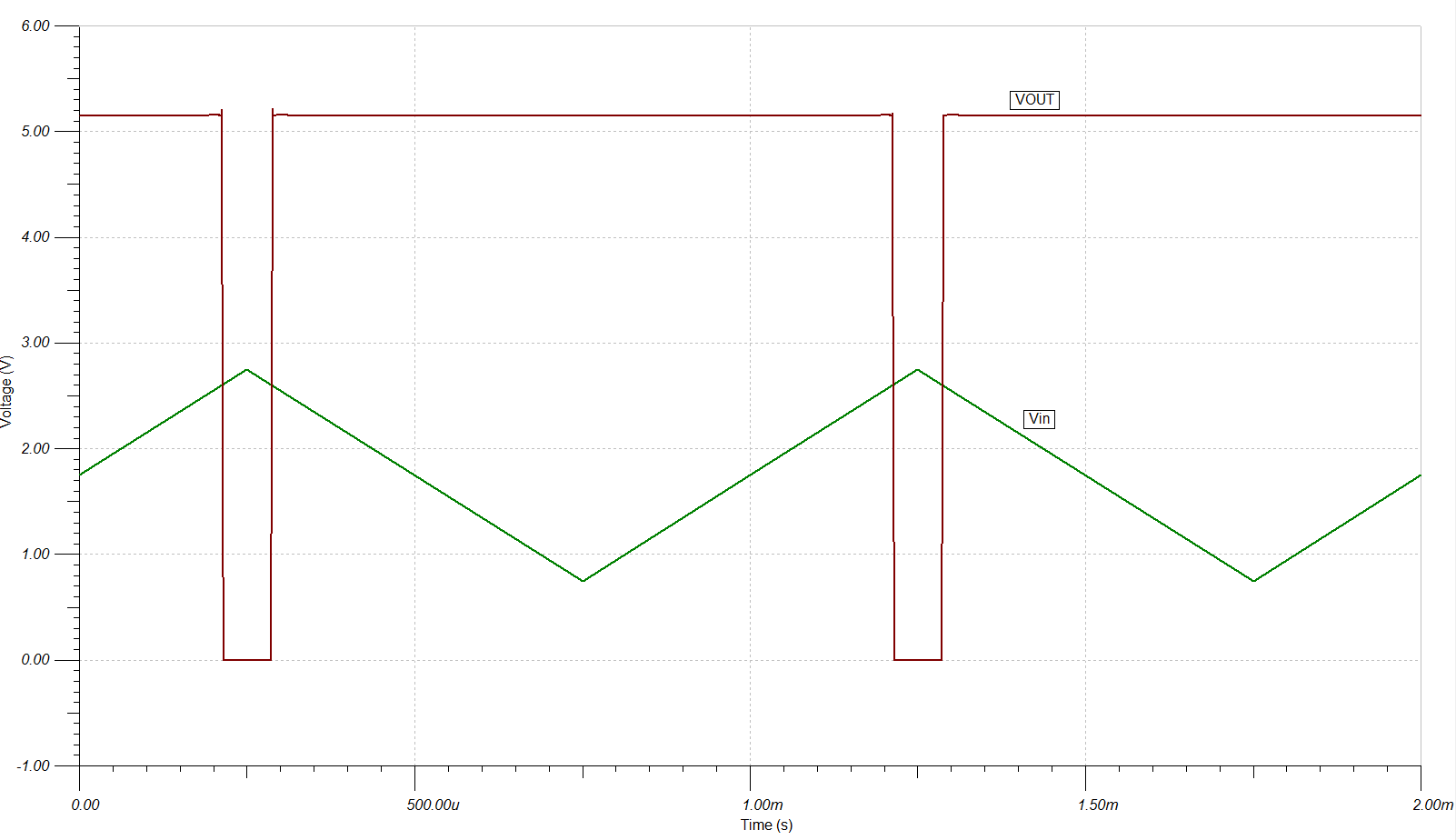Figure 12. Basic Comparator Response

#### 8.2.2 System Examples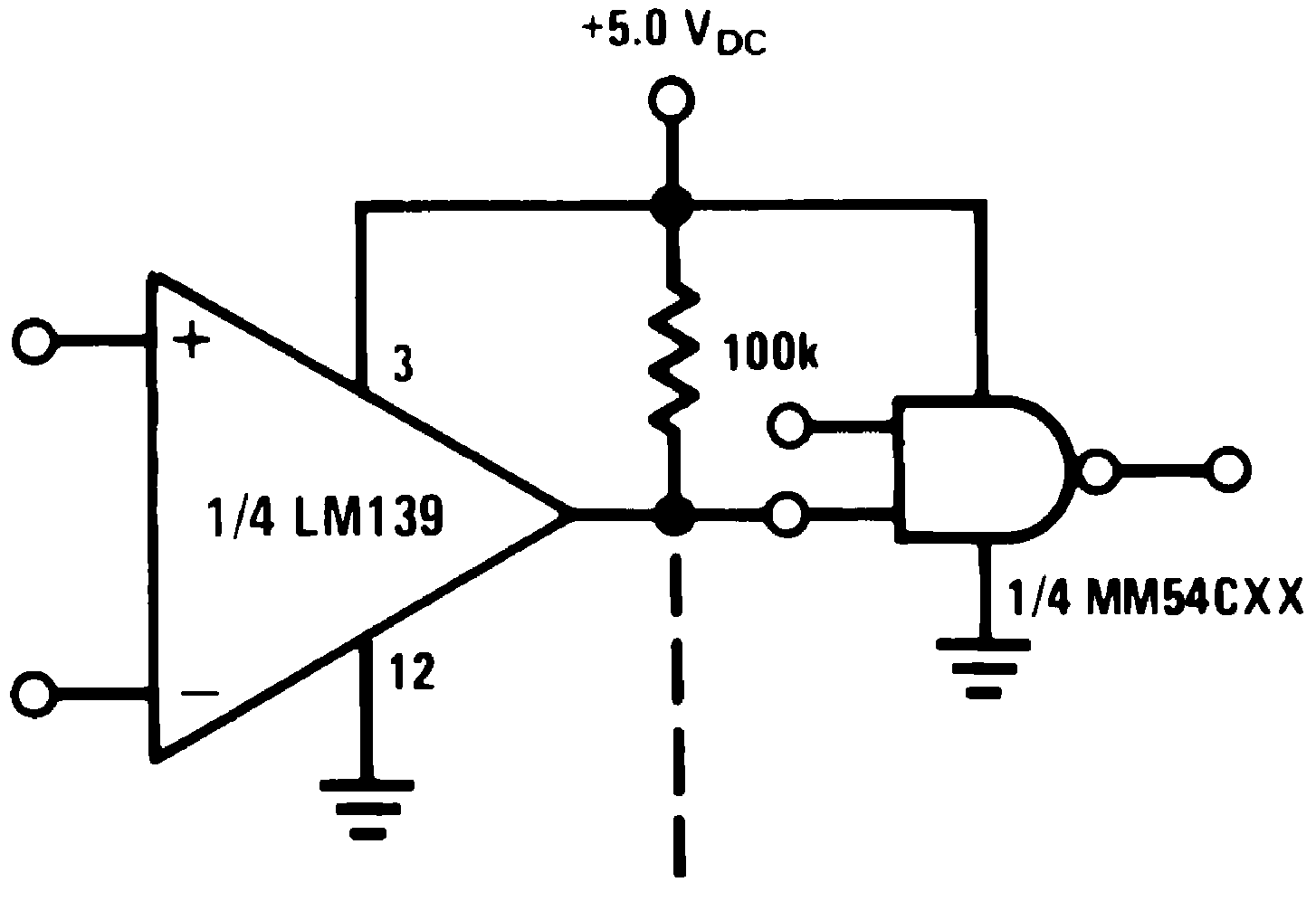Figure 13. Driving CMOS
(V+ = 5.0 VDC)Figure 15. AND Gate
(V+ = 5.0 VDC)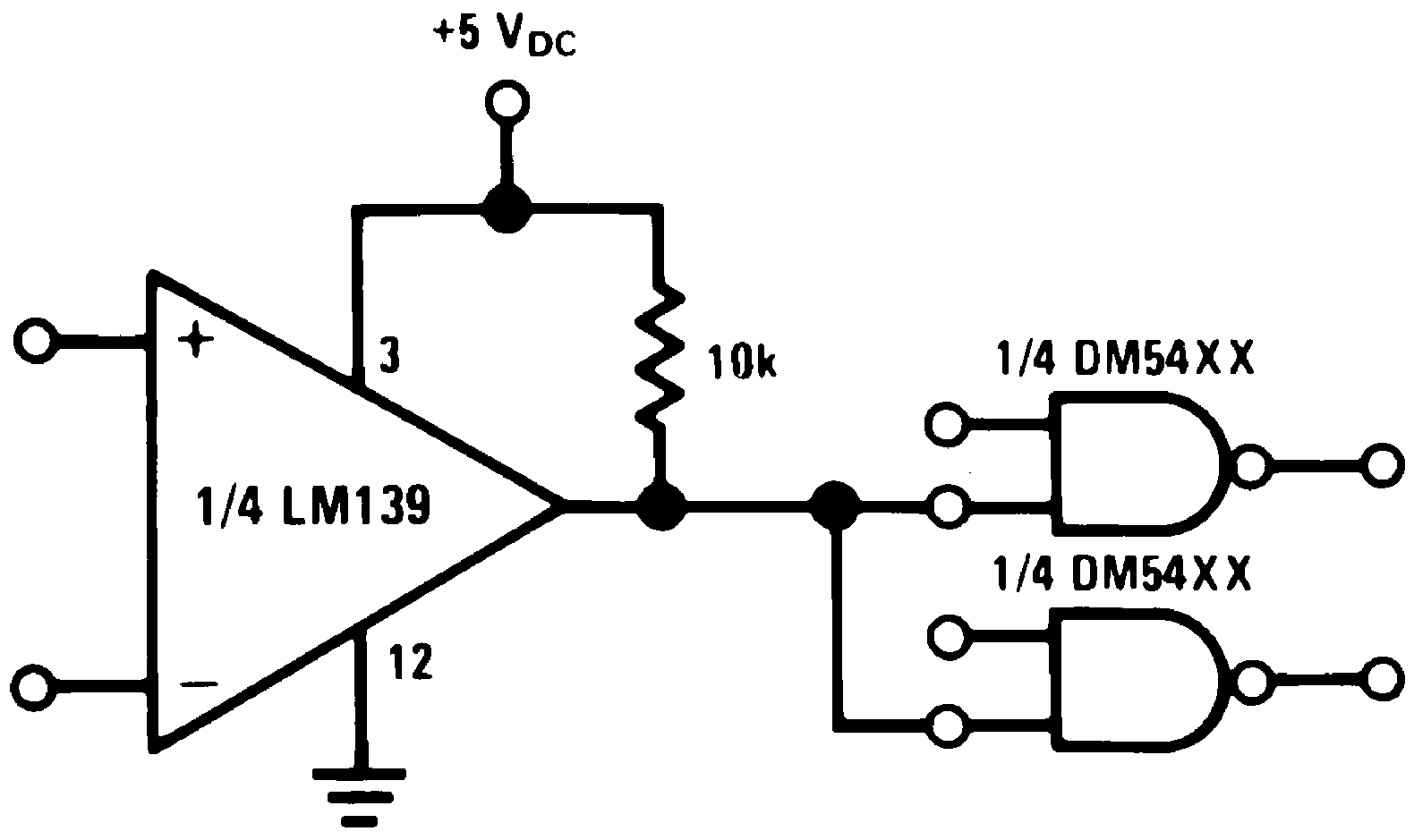Figure 14. Driving TTL
(V+ = 5.0 VDC)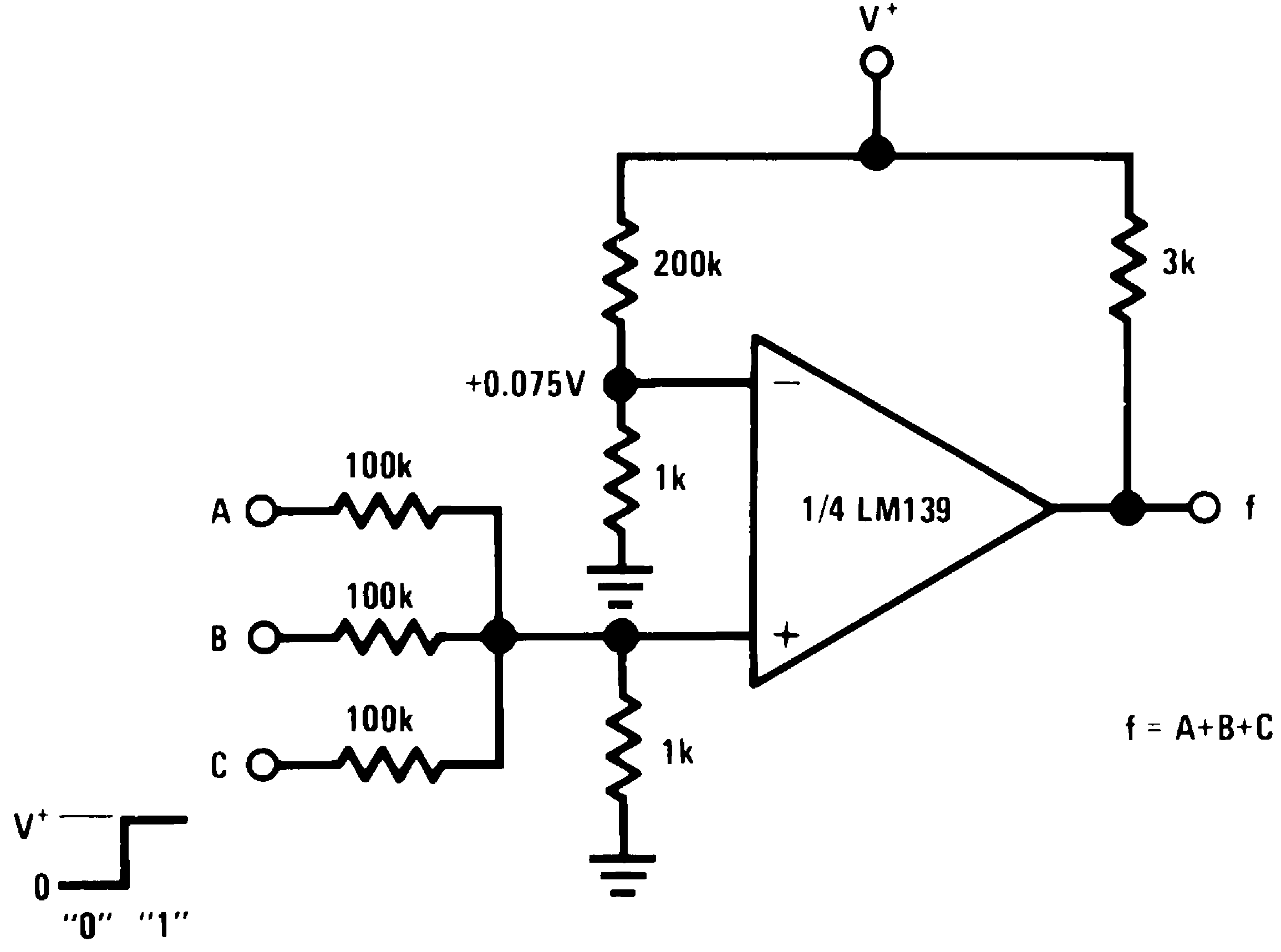Figure 16. OR Gate
(V+ = 5.0 VDC)Figure 17. One-Shot Multivibrator
(V+= 15 VDC)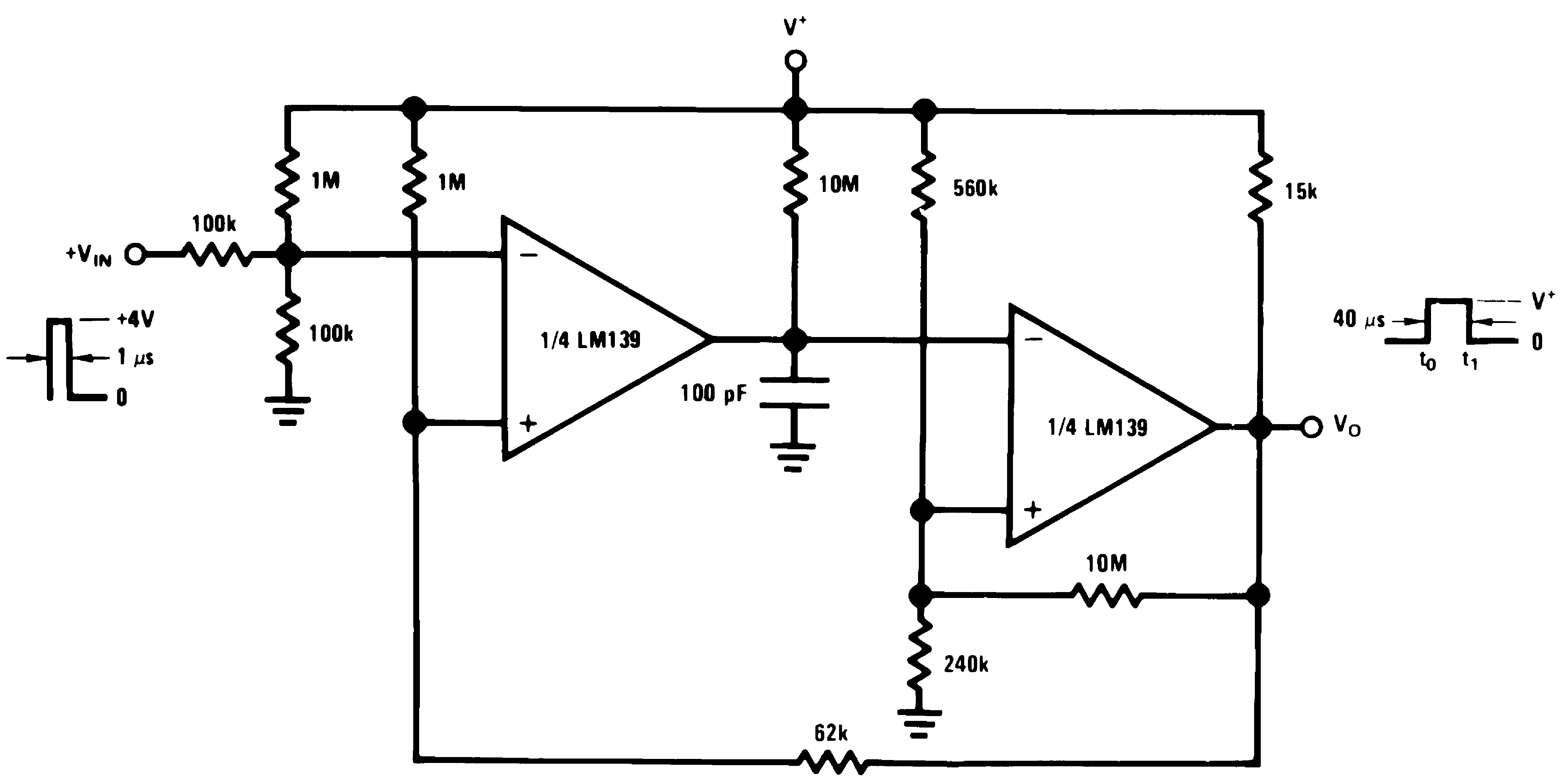Figure 19. One-Shot Multivibrator with Input Lock Out
(V+= 15 VDC)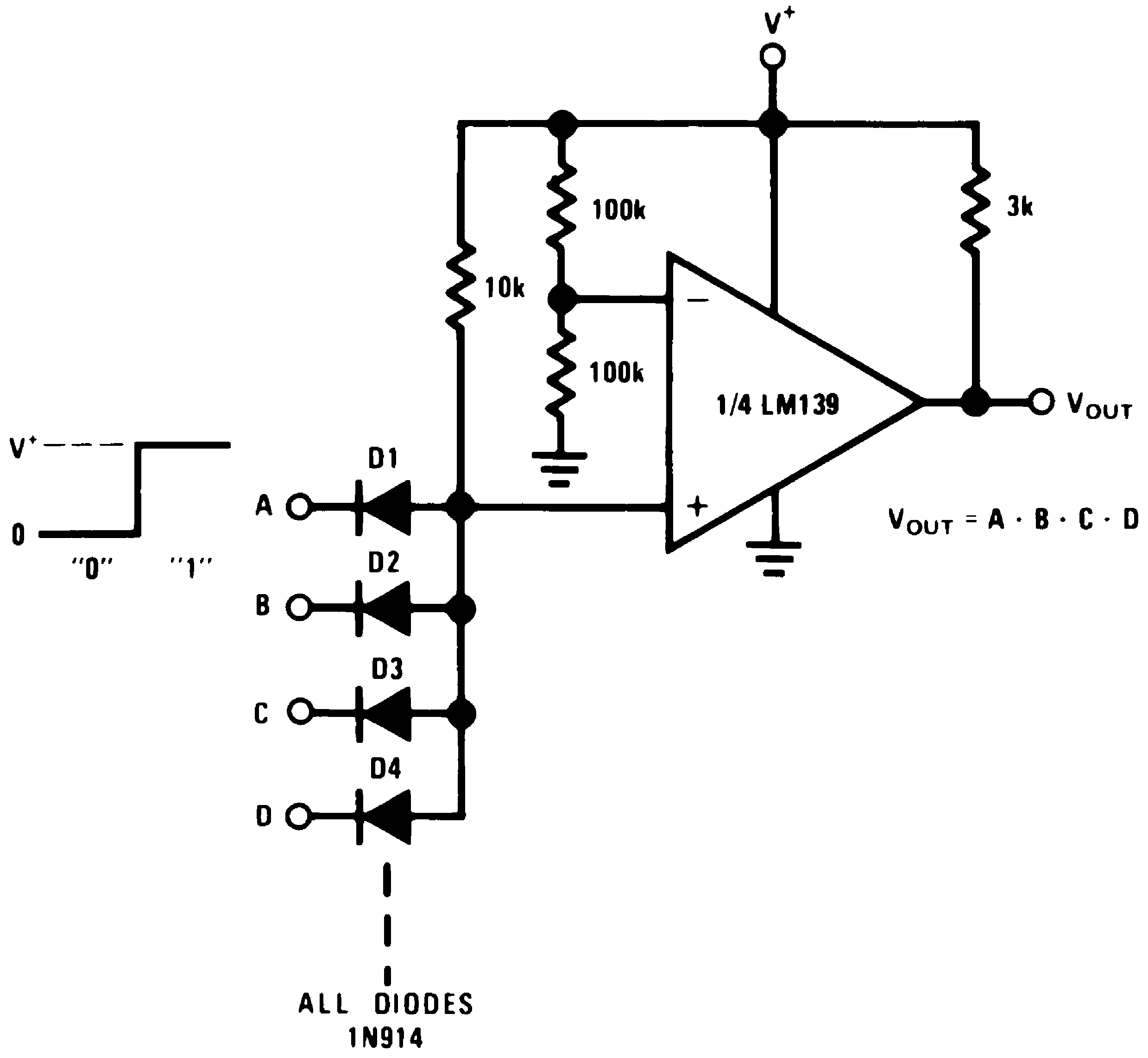Figure 21. Large Fan-In AND Gate
(V+= 15 VDC)Figure 23. Time Delay Generator
(V+= 15 VDC)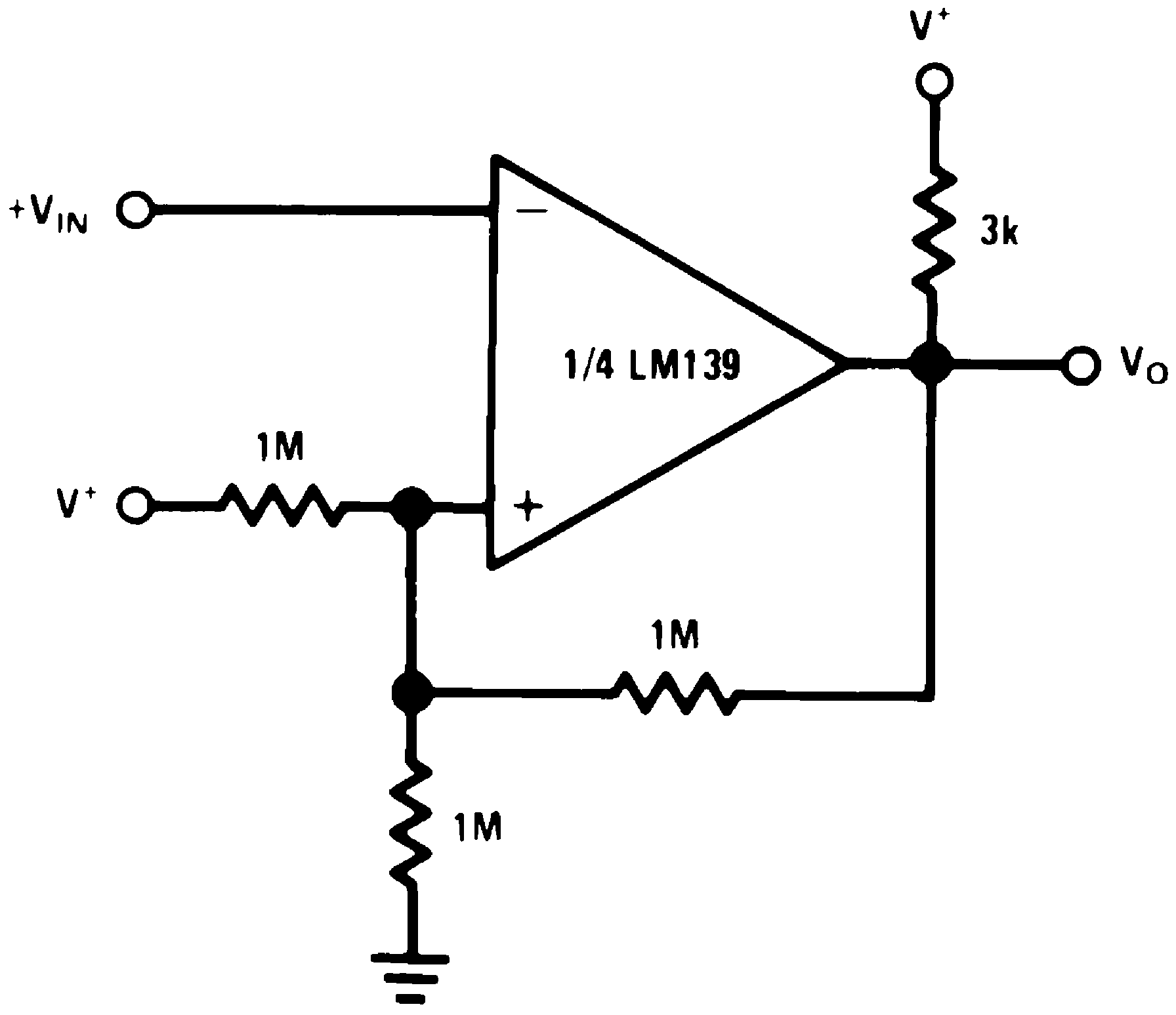Figure 25. Inverting Comparator With Hysteresis
(V+= 15 VDC)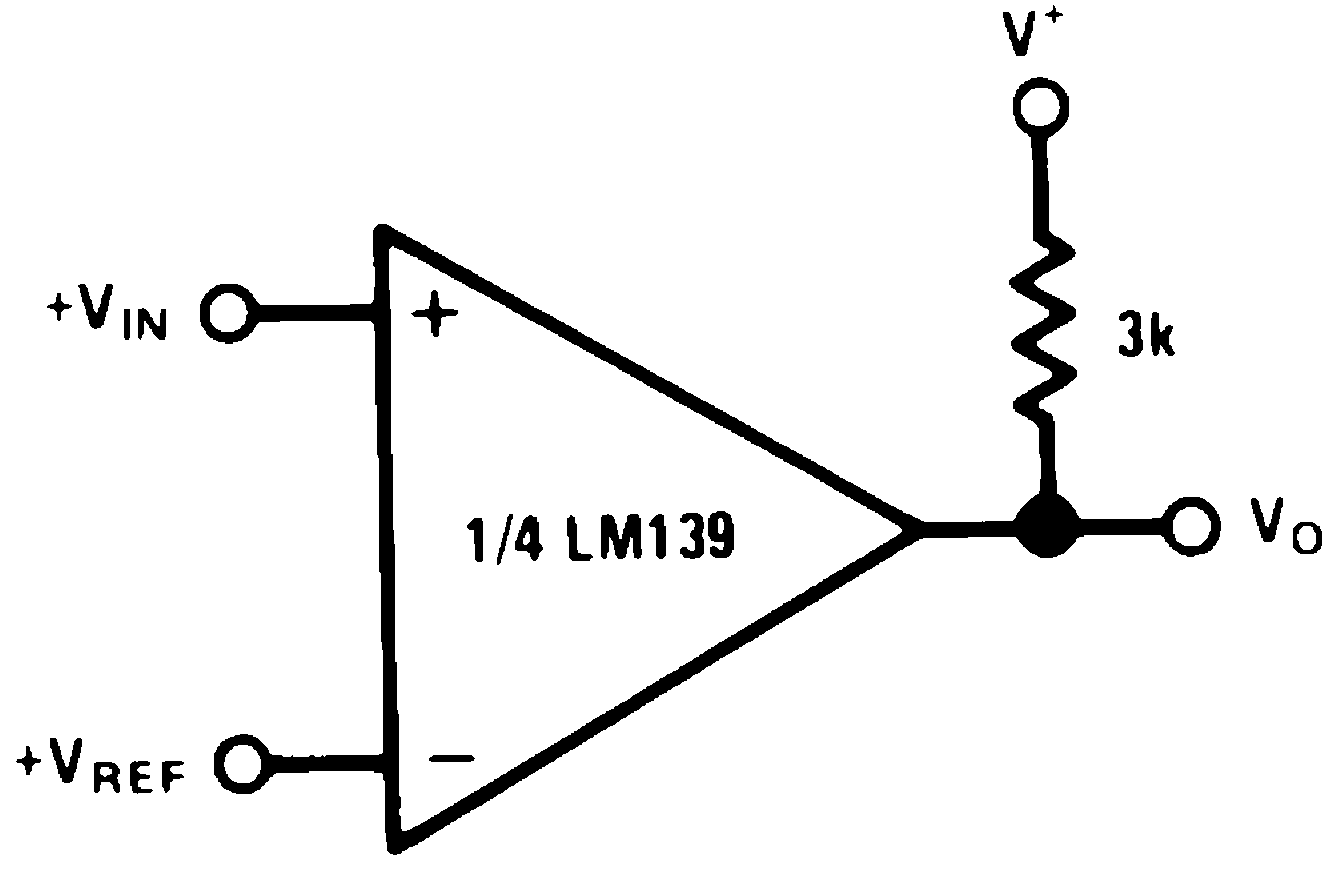Figure 27. Basic Comparator
(V+= 15 VDC)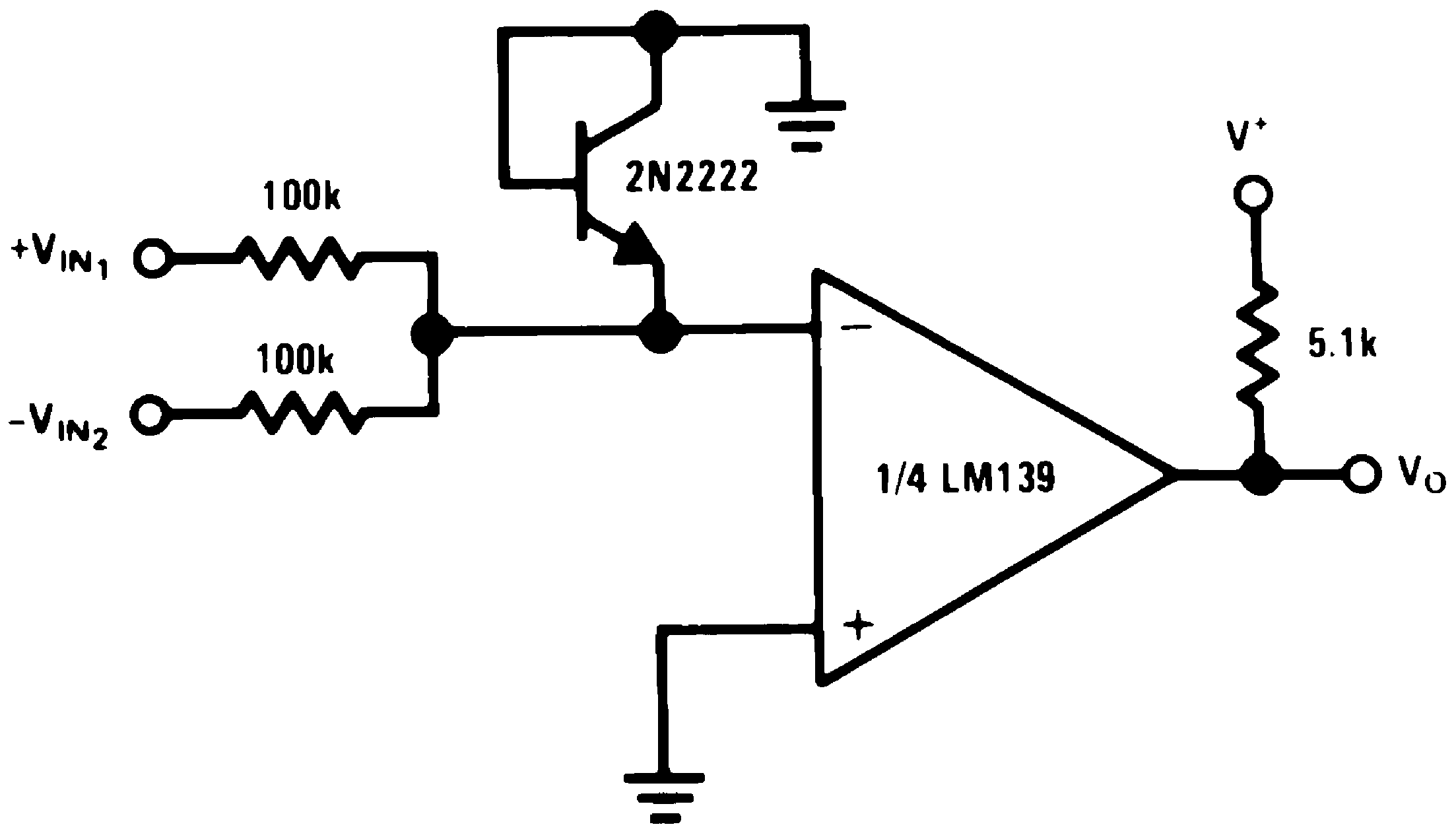Figure 29. Comparing Input Voltages of Opposite Polarity
(V+= 15 VDC)Figure 18. Bi-Stable Multivibrator
(V+= 15 VDC)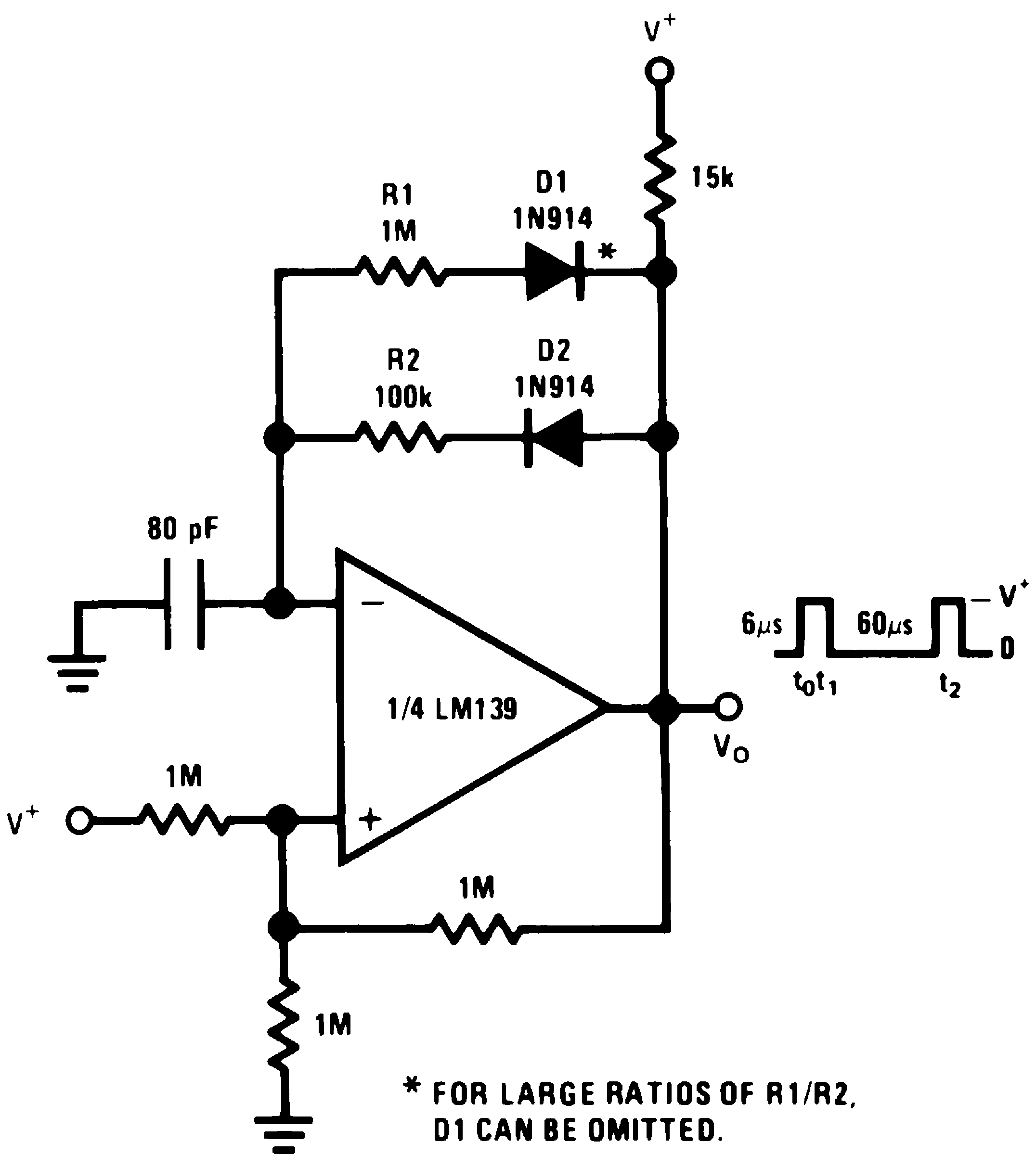Figure 20. Pulse Generator
(V+= 15 VDC)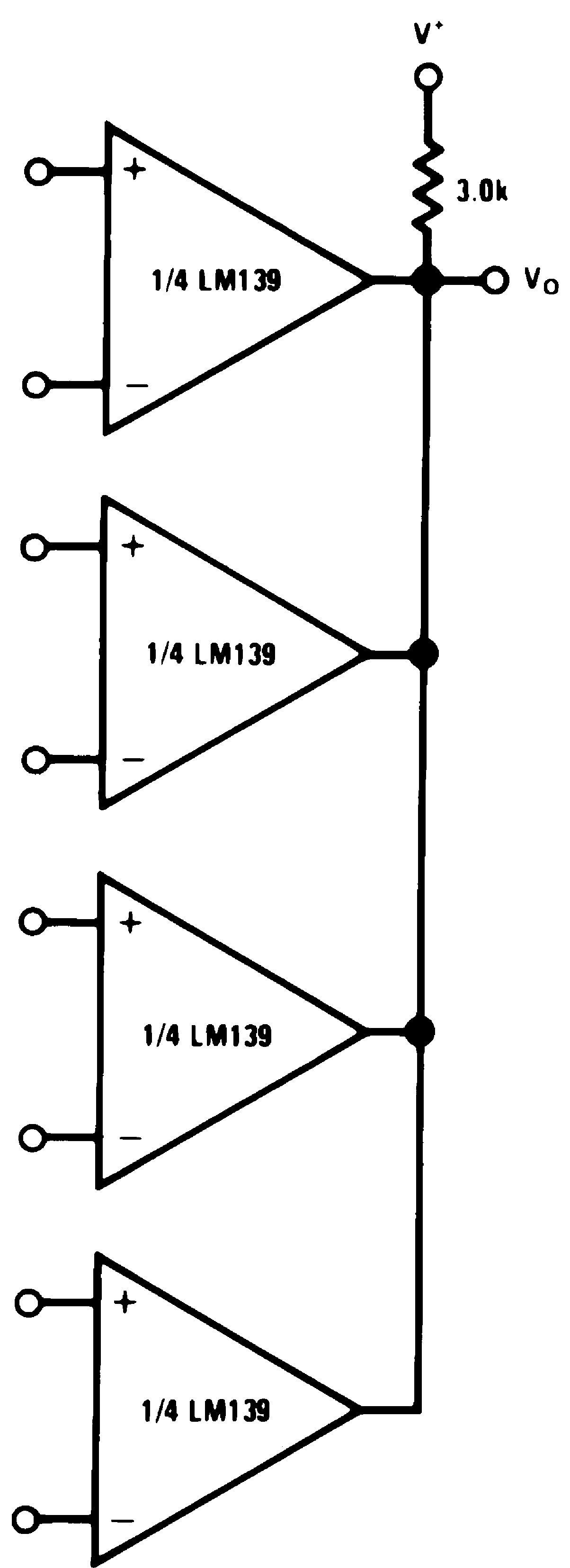Figure 22. ORing the Outputs
(V+= 15 VDC)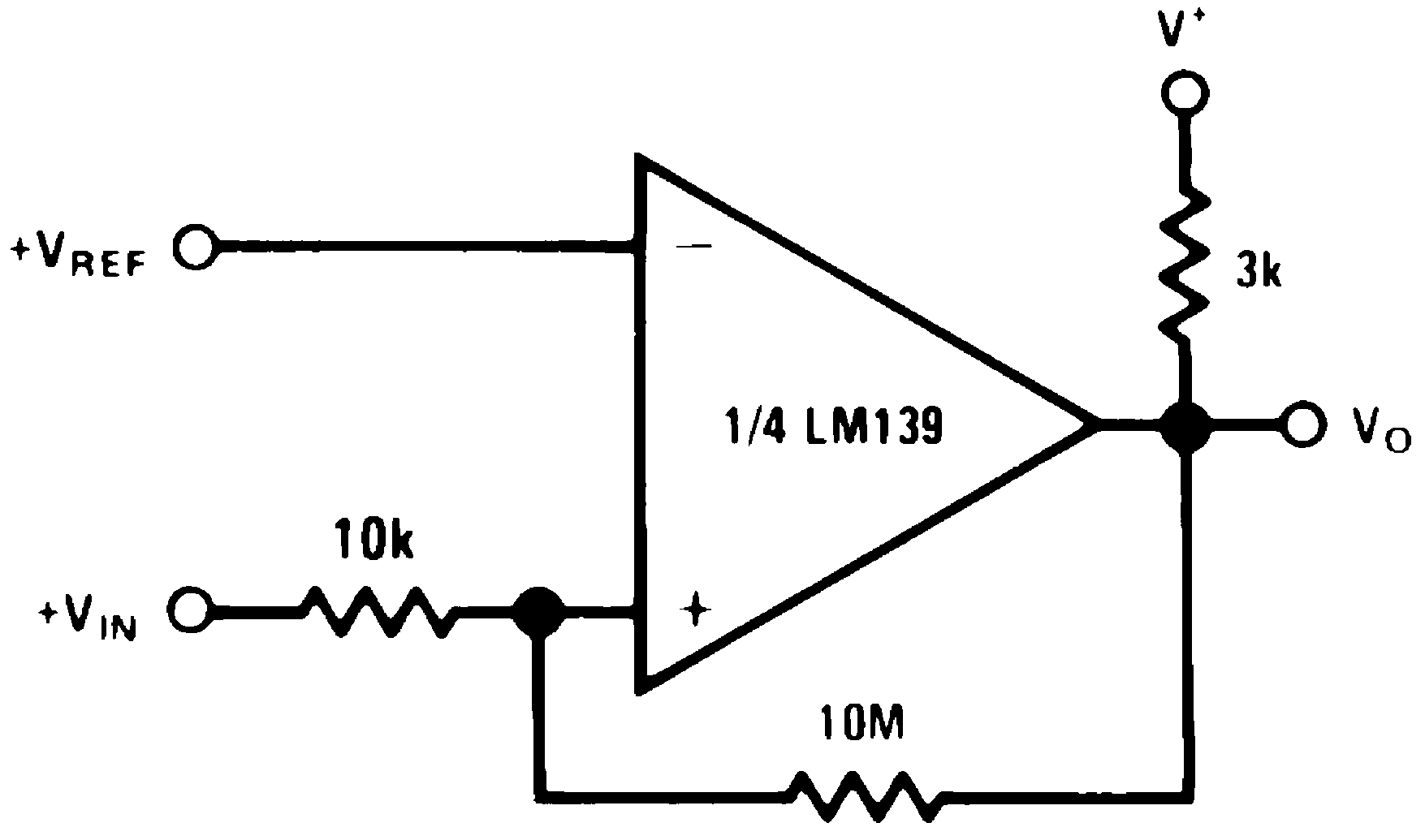Figure 24. Non-Inverting Comparator with Hysteresis
(V+= 15 VDC)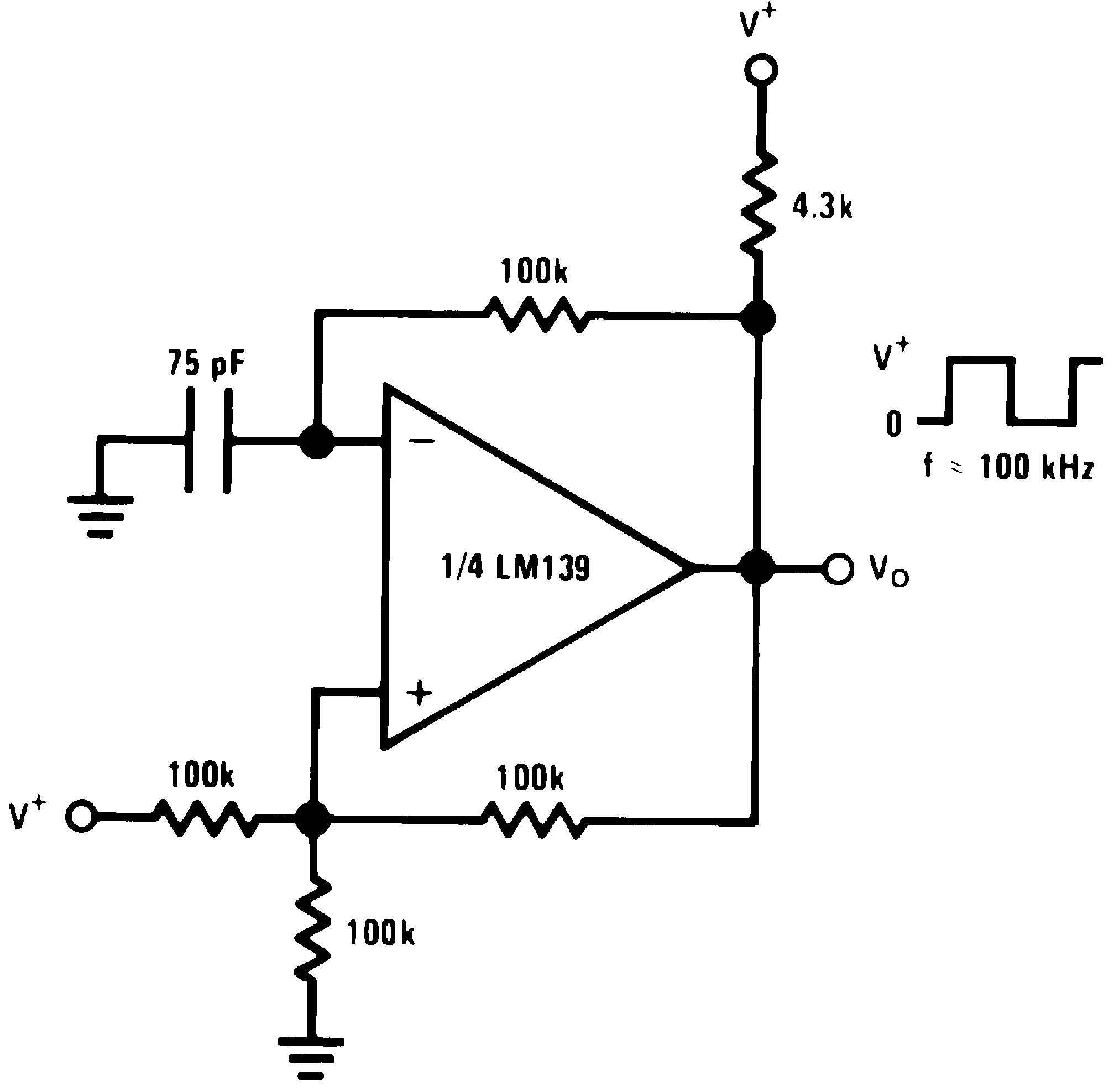Figure 26. Squarewave Oscillator
(V+= 15 VDC)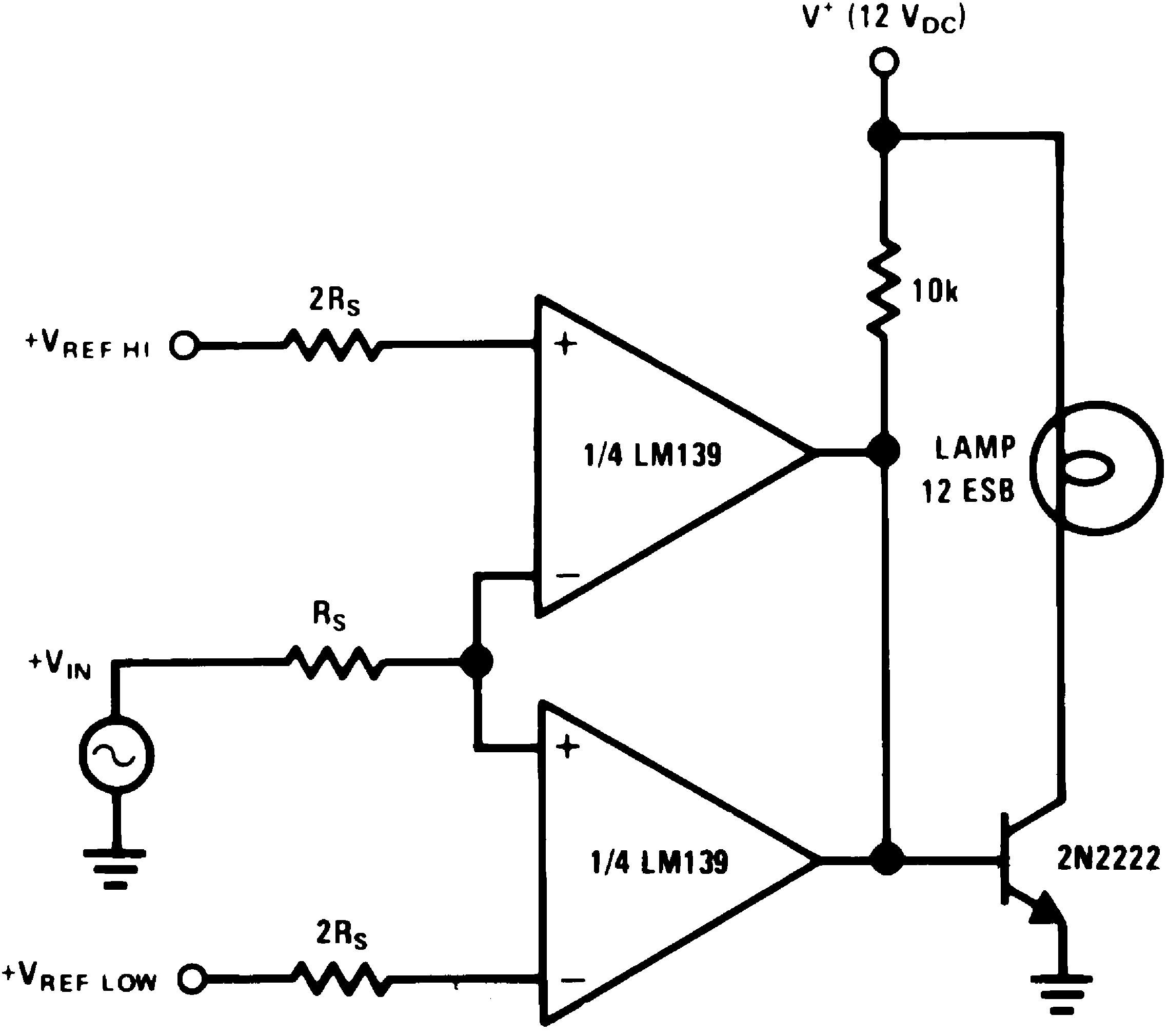Figure 28. Limit Comparator
(V+= 15 VDC)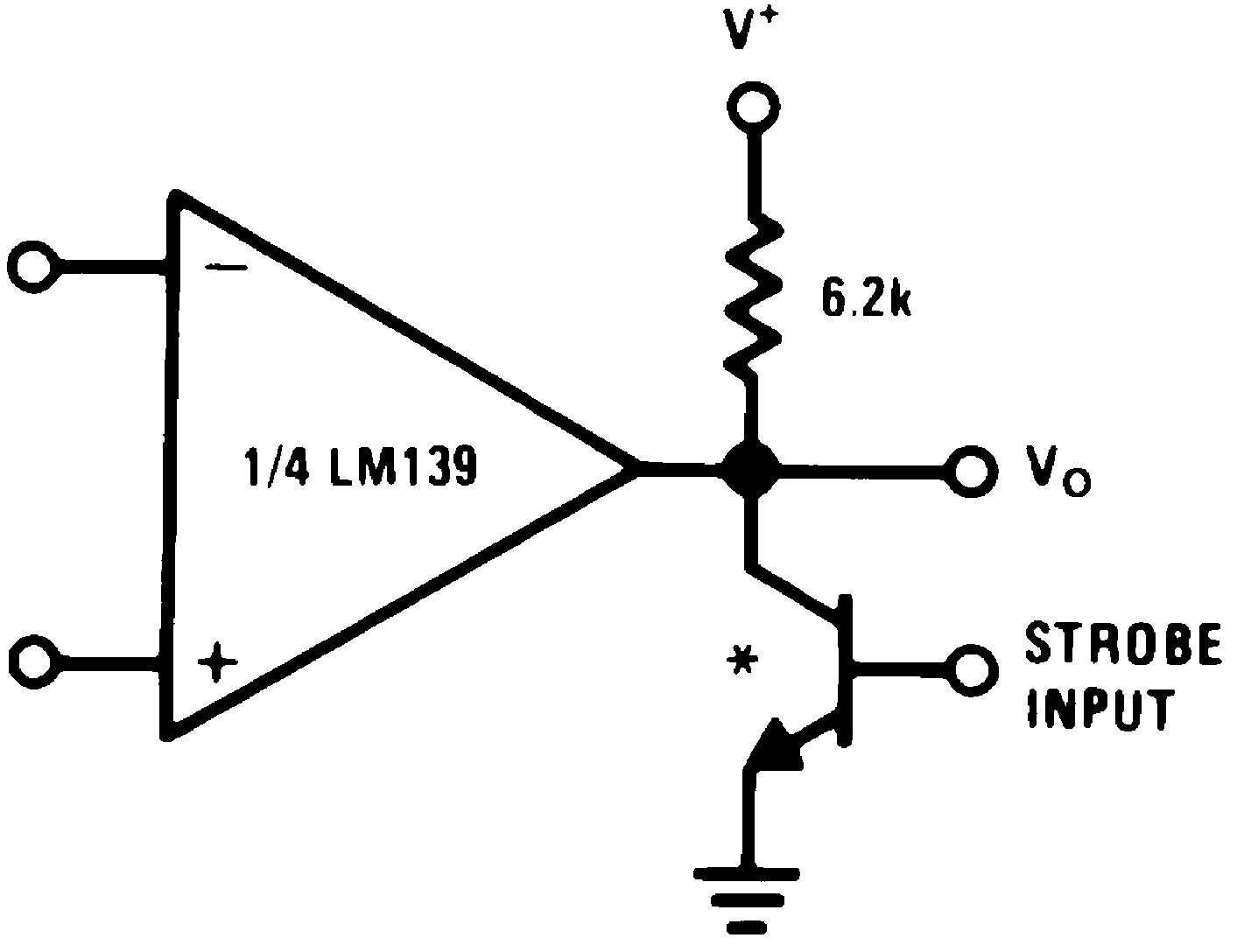* Or open-collector logic gate without pullup resistor
Figure 30. Output Strobing
(V+= 15 VDC)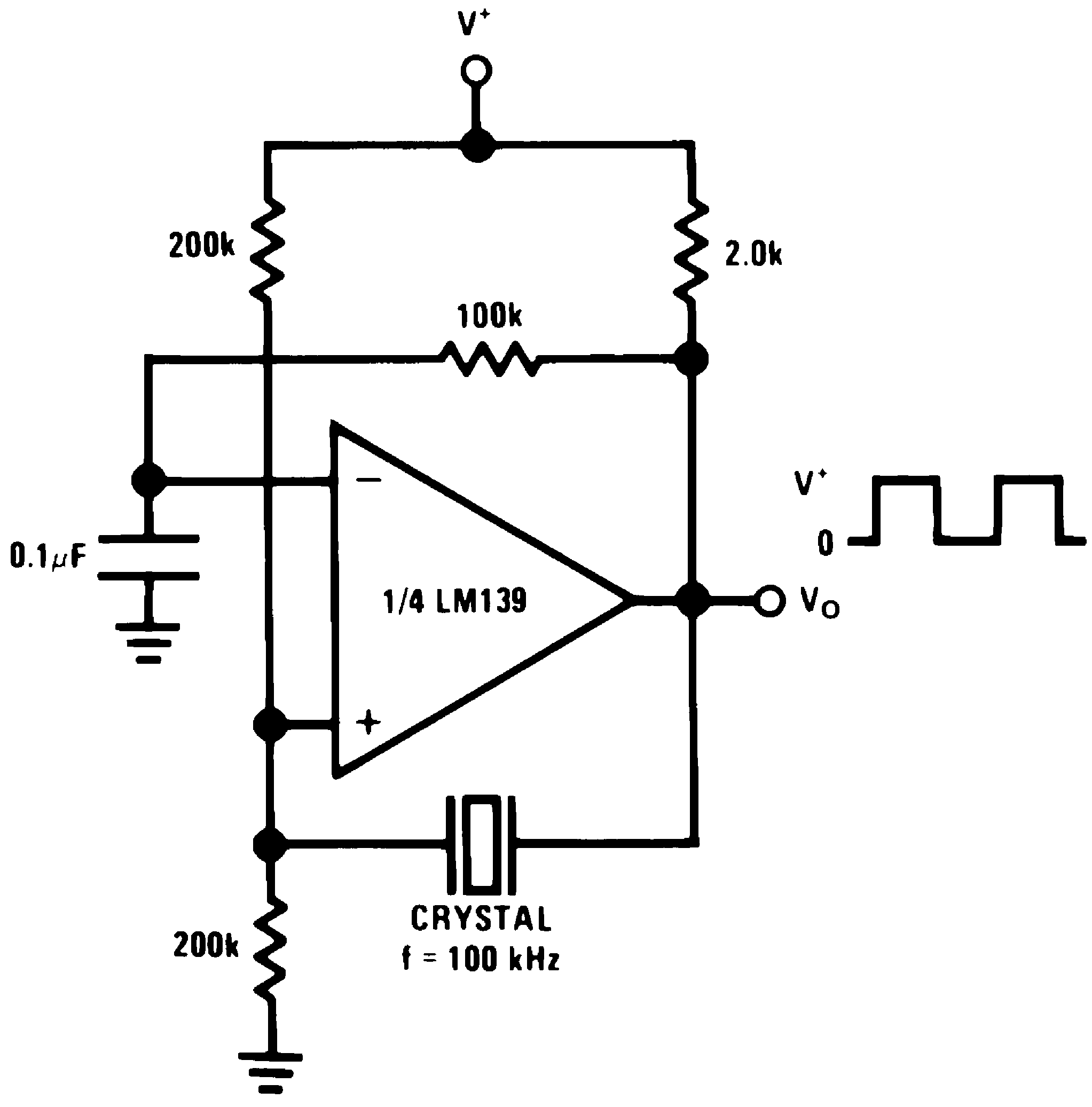Figure 31. Crystal Controlled Oscillator
(V+= 15 VDC)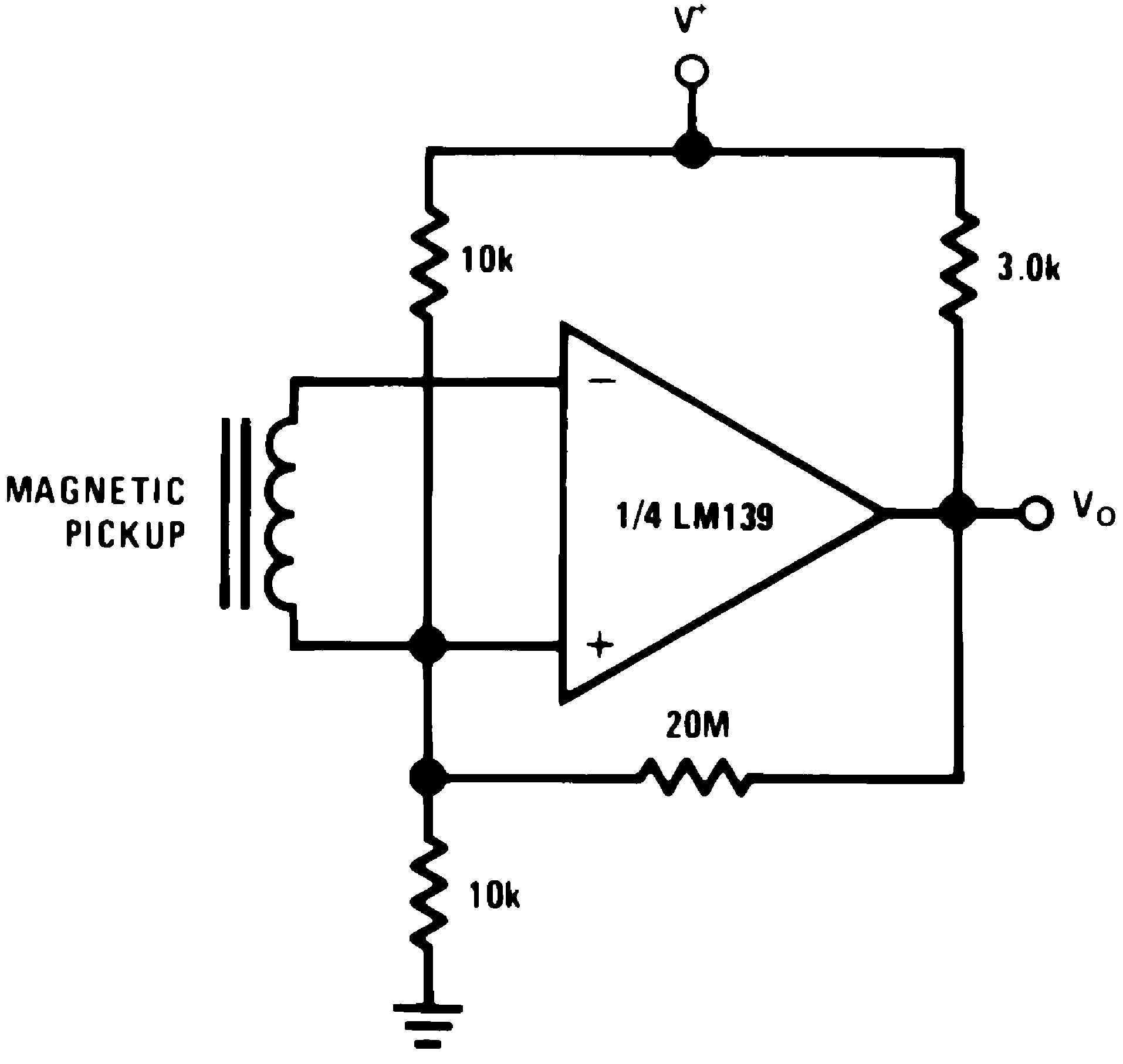Figure 33. Transducer Amplifier
(V+= 15 VDC)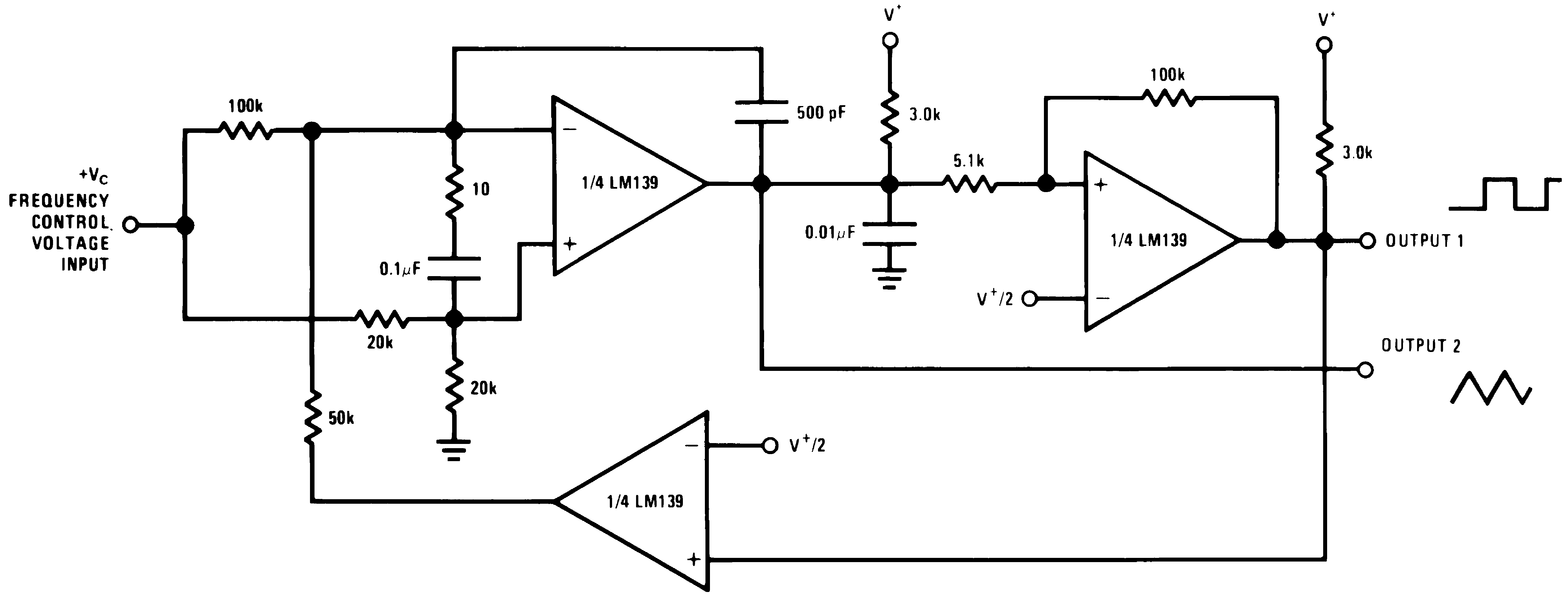250 mVDC ≤ VC ≤ +50 VDC 700 Hz ≤ fO ≤ 100 kHz
V+ = +30 VDC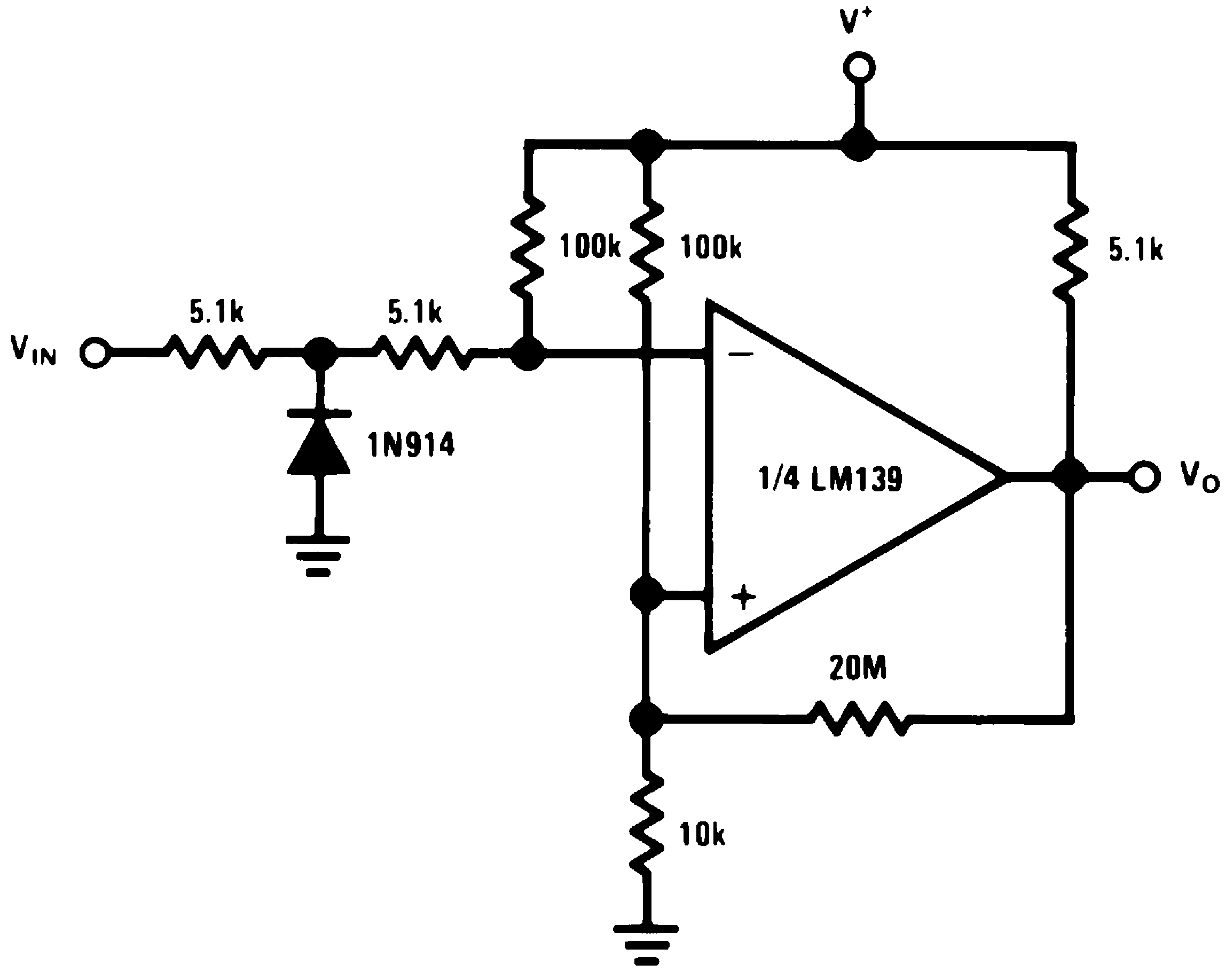Figure 34. Zero Crossing Detector (Single Power Supply)
(V+= 15 VDC)

#### 8.2.2.1 Split-Supply Applications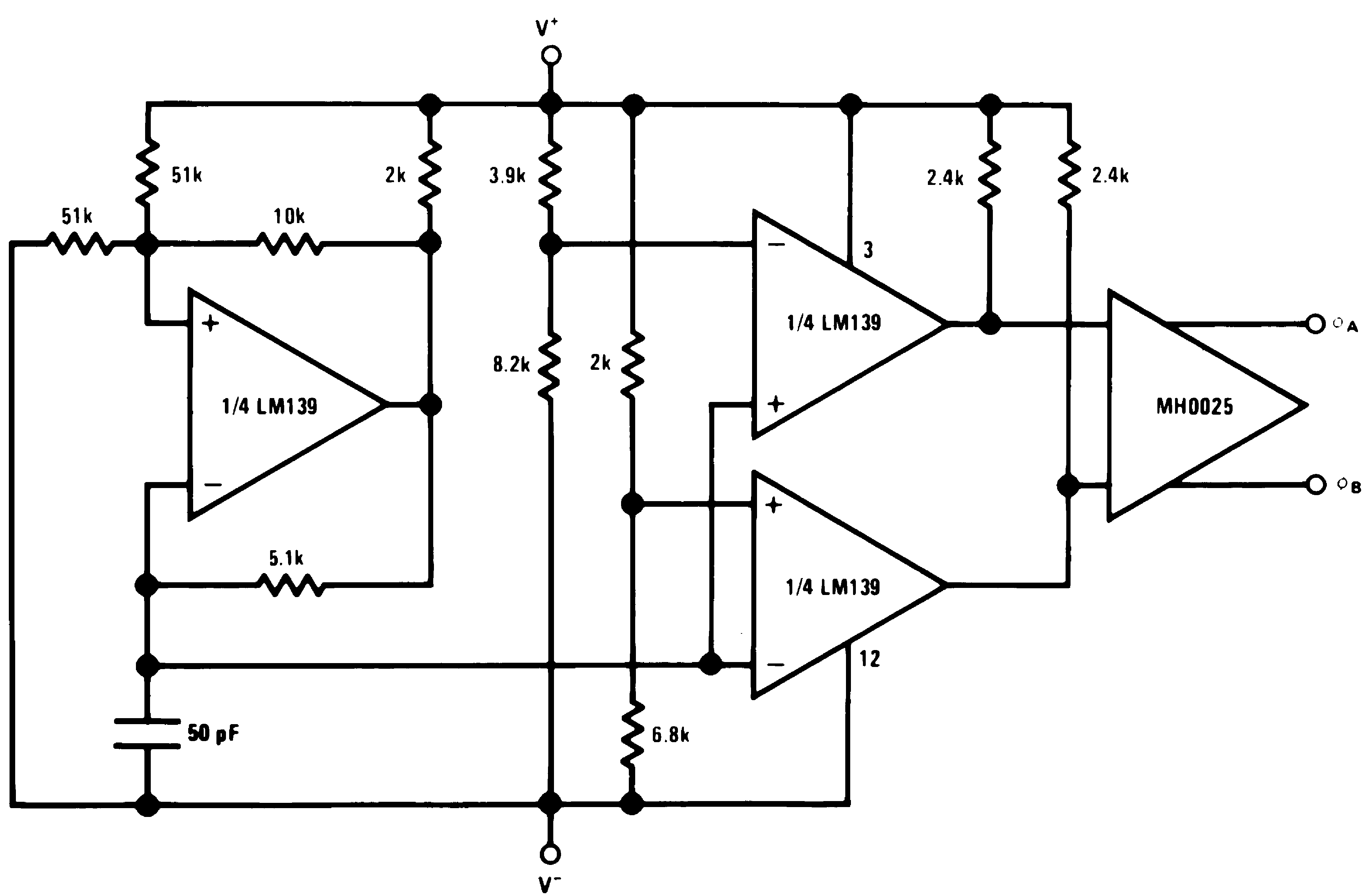Figure 35. MOS Clock Driver
(V+ = +15 VDC and V = −15 VDC)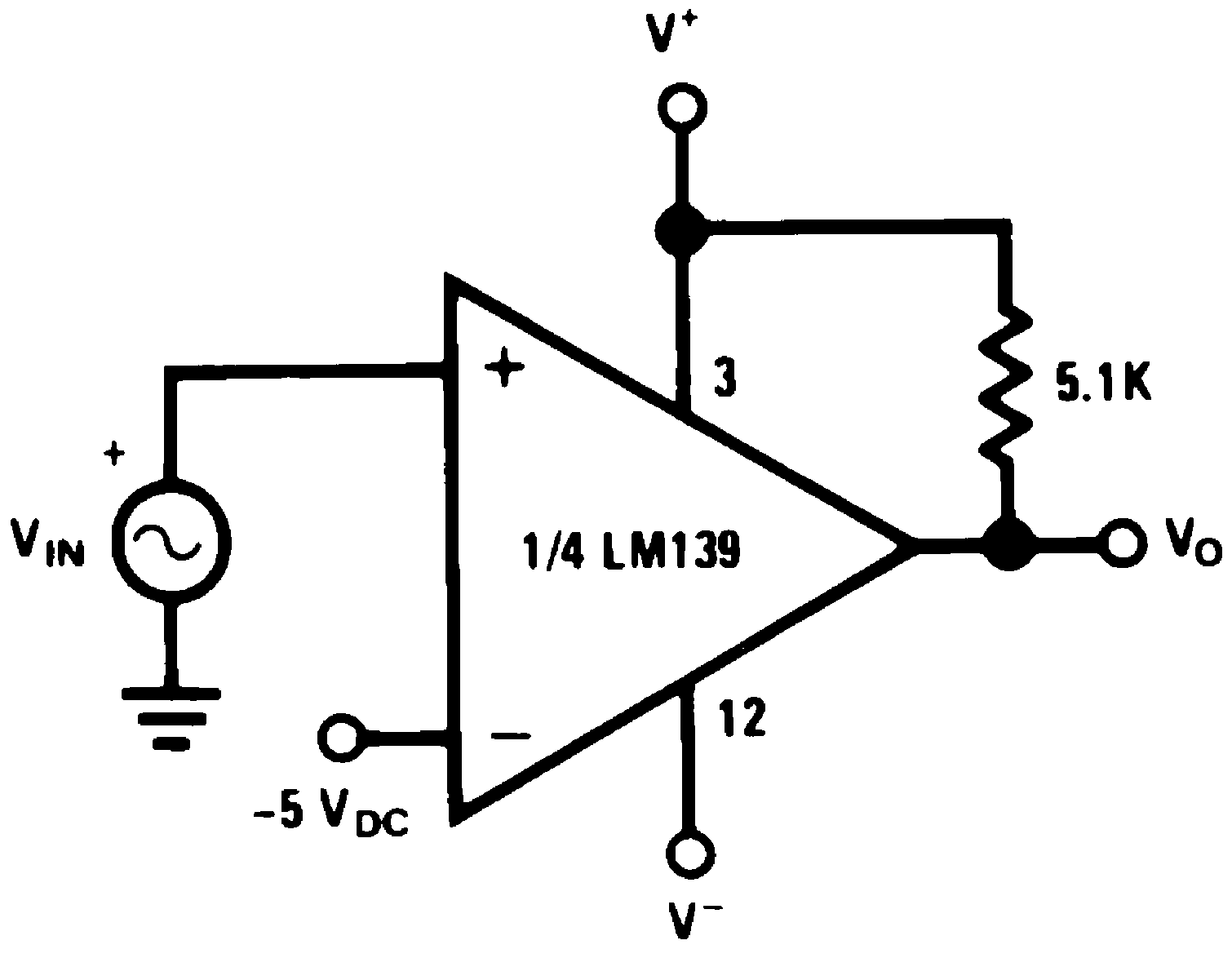Figure 37. Comparator With a Negative Reference
(V+ = +15 VDC and V = −15 VDC)Figure 36. Zero Crossing Detector
(V+ = +15 VDC and V = −15 VDC)